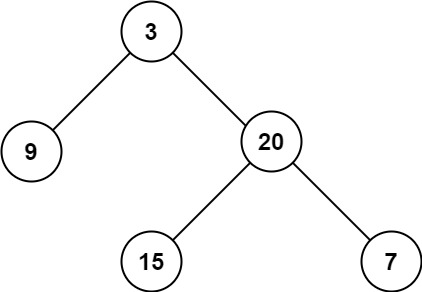| English | 简体中文 |

# 104. Maximum Depth of Binary Tree

## Description

Given the root of a binary tree, return its maximum depth.

A binary tree's maximum depth is the number of nodes along the longest path from the root node down to the farthest leaf node.

Example 1:Input: root = [3,9,20,null,null,15,7]
Output: 3


Example 2:

Input: root = [1,null,2]
Output: 2


Example 3:

Input: root = []
Output: 0


Example 4:

Input: root = 
Output: 1


Constraints:

• The number of nodes in the tree is in the range [0, 104].
• -100 <= Node.val <= 100